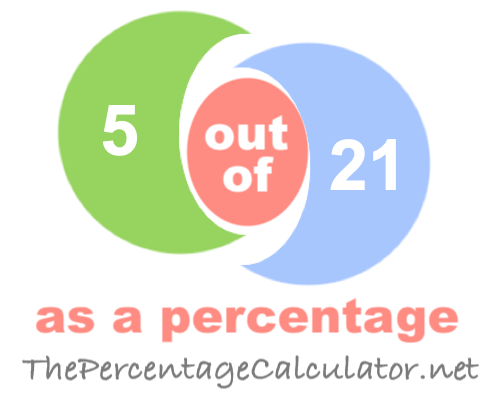What is 5 out of 21 as a percentage?When you ask "What is 5 out of 21?" you want to know what percent 5 is out of 21.

Here are step-by-step instructions showing you how we calculated 5 out of 21 as a percentage:

The first step is to divide 5 by 21 to get the answer in decimal form:

5 ÷ 21 ≈ 0.2381

Then, we multiplied the answer from the first step by one hundred to get the answer as a percentage:

0.2381 × 100 = 23.81%

We can prove that the answer is correct by taking 23.81 percent of 21 to get 5:

(21 × 23.81) ÷ 100 ≈ 5

Note that our calculator rounds the answers up to two decimals if necessary. Once again, the answer is as follows:

≈ 23.81%

Out of as a Percentage Calculator
To solve another problem, please submit it below:

out of

What is 5 out of 22 as a percentage?
The solution to "What is 5 out of 21 as a percentage?" is not the only answer we have. Go here for the next solution on our list.